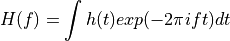# 11.10.1. astroML.fourier.FT_continuous¶

astroML.fourier.FT_continuous(t, h, axis=- 1, method=1)[source]

Approximate a continuous 1D Fourier Transform with sampled data.

This function uses the Fast Fourier Transform to approximate the continuous fourier transform of a sampled function, using the conventionIt returns f and H, which approximate H(f).

Parameters
tarray_like

regularly sampled array of times t is assumed to be regularly spaced, i.e. t = t0 + Dt * np.arange(N)

harray_like

real or complex signal at each time

axisint

axis along which to perform fourier transform. This axis must be the same length as t.

Returns
fndarray

frequencies of result. Units are the same as 1/t

Hndarray

Fourier coefficients at each frequency.